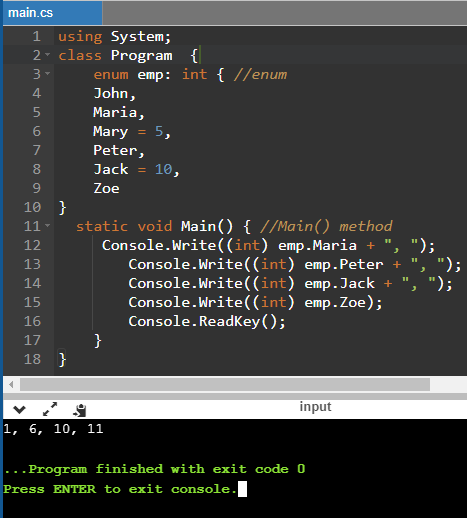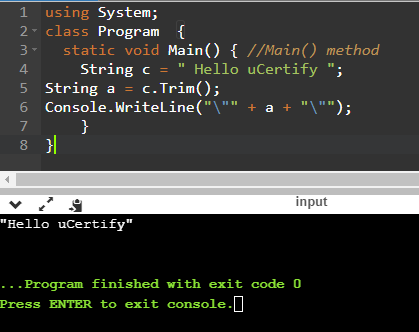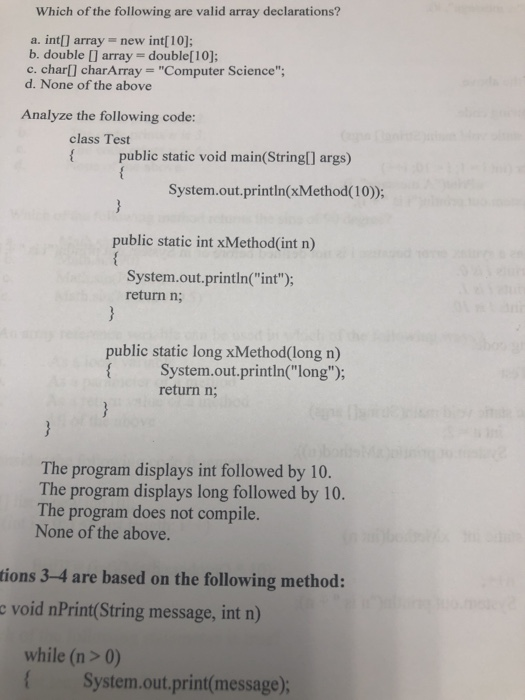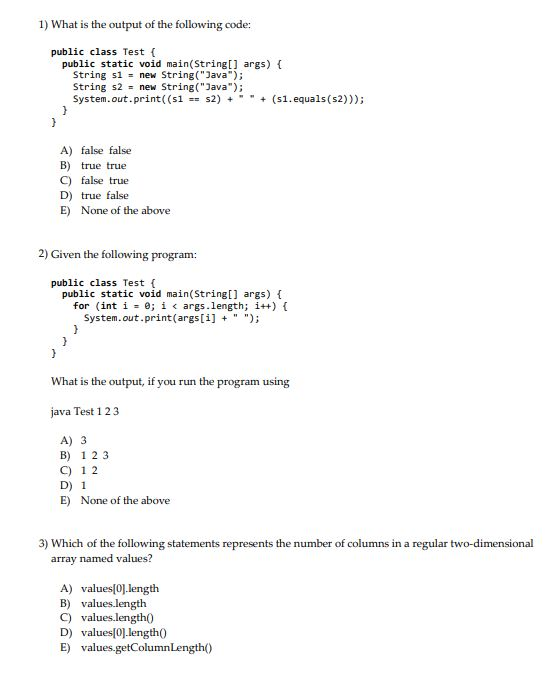Question

# Which of the following is responsible for the execution of all applications developed using the .NET...

Which of the following is responsible for the execution of all applications developed using the .NET library?

A

Common Type System

B

Base Class Libraries

C

Common Intermediate Language

D

Common Language Runtime

What is a function of the Solution Explorer window in Visual Studio?

This task contains the radio buttons and checkboxes for options. The shortcut keys to perform this task are A to H and alt+1 to alt+9.

A

It shows the hierarchy of files and folders in the project.

B

It lists the errors present in the code.

C

It provides access to the user interface building blocks for desktop applications.

D

It displays information about the current Team Foundation Server.

What will be the output of the following C# program?

```class Program {

enum emp: int {
John,
Maria,
Mary = 5,
Peter,
Jack = 10,
Zoe
}

static void Main(string[] args) {
Console.Write((int) emp.Maria + ", ");
Console.Write((int) emp.Peter + ", ");
Console.Write((int) emp.Jack + ", ");
Console.Write((int) emp.Zoe);
}
}```

A

1, 6, 10, 11

B

2, 6, 7, 8

C

2, 6, 10, 11

D

1, 6, 10, 8

What will be the output of the following code?

` `
1. static void Main(string[] args) {
2. String c = " Hello uCertify ";
3. String a = c.Trim();
4. Console.WriteLine("\"" + s + "\"");
5. }

A

"Hello uCertify"

B

" Hello uCertify"

C

"HellouCertify"

D

Hello uCertify

Dear Student ,

As per requirement submitted above kindly find below solution.

Question 1:

Explanation :

• Common Language Runtime (CLR) provide all the resources required to run a dot net application within  .net environment.
• Common Type System is a collection of all data types in the ,net.
• Base Class Libraries is collection of all inbuilt classed in .net which approximately contains 6000 classes , 65000 methods , 1 lakh properties.
• Common Intermediate Language (CIL) is the byte code and which is the common language of the .net application.

****************************

Question 2:

Answer :A.It shows the hierarchy of files and folders in the project.

Explanation :Solution explorer in visual studio contains all local files and folder along with configuration files and images.

****************************

Question 3:

Answer :A 1, 6, 10, 11

Explanation :Enum member in C# can have the value of one incremented of previous member , if previous member is not present then value starts with 0.

Below screen shows the output****************************

Question 4:

Explanation :

• Will get the output above by ignoring the error in the line Console.WriteLine("\"" + a + "\""); here it is a not s
• Below screen shows the output****************************

#### Earn Coins

Coins can be redeemed for fabulous gifts.

Similar Homework Help Questions
• ### ==Modify the M8B by using C#== using System; using System.Collections.Generic; using System.Linq; using System.Text; using System.Threading.Tasks;...

==Modify the M8B by using C#== using System; using System.Collections.Generic; using System.Linq; using System.Text; using System.Threading.Tasks; namespace M8B {     class Magic8Ball     {         static void Main(string[] args)         {             string question;             Console.WriteLine("I am the Magic 8-ball! Ask me a question and I will give you an answer.");             Console.Write("Your question: ");             question = Console.ReadLine();             Console.WriteLine("\nLet me part the mists of the time for you.");             Random rnd = new Random();             int roll =...

• ### Hello in C#. I need help understanding and knwoing what i missed in my program. The...

Hello in C#. I need help understanding and knwoing what i missed in my program. The issue is, the program is supposed to have user input for 12 family members and at the end of the 12 it asks for user to input a name to search for. When i put a correct name, it always gives me a relative not found message. WHat did i miss. And can you adjust the new code so im able to see where...

• ### C#, I am getting error placing them all in one file pls set them in the...

C#, I am getting error placing them all in one file pls set them in the order that all 3 work in one file. Thanks //Program 1 using System; class MatrixLibrary { public static int[,] Matrix_Multi(int[,] a, int [,]b){ int r1= a.GetLength(0); int c1 = a.GetLength(1); int c2 = b.GetLength(1); int r2 = b.GetLength(0); if(r2 != c2){ Console.WriteLine("Matrices cannot be multiplied together.\n"); return null;    }else { int[,] c = new int[r1, c2]; for (int i = 0; i <...

• ### i need help fixing my code. here is the assignment: Make a class that contains the...

i need help fixing my code. here is the assignment: Make a class that contains the following functions: isLong, isDouble, stringToLong, and stringToDouble. Each receives a string and returns the appropriate type (bool, bool, long, and double).During the rest of the semester you will paste this class into your programs and will no longer use any of the "standard" c# functions to convert strings to numbers. here is my code. it works for the long method but not the double:...

• ### Which of the following are valid array declarations? a. int[] array- new int; b. double [array...Which of the following are valid array declarations? a. int[] array- new int; b. double [array double; c. charl charArray "Computer Science"; None of the above Analyze the following code: class Test public static void main(Stringl] args) System.out.println(xMethod(10); public static int xMethod(int n) System.out.println("int"); return n; public static long xMethod(long n) System.out.,println("long"); return n The program displays int followed by 10 The program displays long followed by 10. The program does not compile. None of the above. tions 3-4 are...

• ### 1) Consider the following Java program: 1 public class HelloWorld { 2     // My first program!...

1) Consider the following Java program: 1 public class HelloWorld { 2     // My first program! 3     public static void main(String[] args) { 4         System.out.println("Hello, World!"); 5     } 6 } What is on line 1? a. a variable declaration b. a statement c. a method (subroutine) definition d. a comment e. a class definition 2) Which one of the following does NOT describe an array? a. It can be used in a for-each loop. b. It has a numbered sequence...

• ### Write a C# program (Integer Math Calculator, the programming requirements are as follows: When typing in...

Write a C# program (Integer Math Calculator, the programming requirements are as follows: When typing in 3+4, 10-5, 12*12, 15/5 from console, the program should give the right answers on screen like 7, 5, 144, 3. This is what I have so far: namespace ConsoleApplication3 { class Program { static void Main(string[] args) { String input; do { Console.Write("Type int values to calulate or stop to exit: "); input = Console.ReadLine(); if (input.ToLower() != "stop") { char[] delimiters = {...

• ### In each of the following questions, first use NetBeans IDE to run the code (if there...

In each of the following questions, first use NetBeans IDE to run the code (if there is an error fix it) then take a screenshot of the output and paste it under (A). In (B), justify the output you obtained in (A). - 9. Given the following: public class WorkingSheet {     public static void main(String[] args) {        B myB = new B();        A myA = new B();        System.out.print(myB instanceof A);        System.out.print(myB instanceof C);        System.out.print(myA...

• ### Language is Java. Thank you.It is a review test and I will find out the rest...Language is Java. Thank you.It is a review test and I will find out the rest if I just know that answers. 1) What is the output of the following code: public class Test public static void main(String] args) [ String s1 new String("Java String s2 new String("Java) System.out.print( (s1 s2)(s1.equals (s2))) A) false false B) true true C) false true D) true false E) None of the above 2) Given the following program: public class Test f public static...